Reach Us+44-1522-440391
On a correspondence between ideals and commutativity in algebraic crossed products 1 | OMICS International
Journal of Generalized Lie Theory and Applications
All submissions of the EM system will be redirected to Online Manuscript Submission System. Authors are requested to submit articles directly to Online Manuscript Submission System of respective journal.

# On a correspondence between ideals and commutativity in algebraic crossed products 1

Johan OINERT* and Sergei D. SILVESTROV

Centre for Mathematical Sciences, Lund University, Box 118, SE-221 00 Lund, Sweden E-mails: [email protected], [email protected]

*Corresponding Author:
Johan OINERT
Centre for Mathematical Sciences,
Lund University, Box 118,
SE-221 00 Lund, Sweden
E-mails: [email protected], [email protected]

Received date: December 16, 2007 Accepted Date: April 01, 2008

Visit for more related articles at Journal of Generalized Lie Theory and Applications

#### Abstract

In this paper we will give an overview of some recent results which display a connection between commutativity and the ideal structures in algebraic crossed products.

#### Introduction

In the recent papers [3,4], we have been studying a correspondence between ideals and commutativity in algebraic crossed products. Given an algebraic crossed product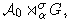, consider the following two statements:

S1: The coefficient ring A0 is a maximal commutative subring in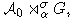S2: For every non-zero two-sided ideal I in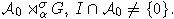In this paper we will give an overview of some types of crossed products for which the statements S1 and S2 are equivalent. We will also give an example of a situation in which these statements are not equivalent. For a general crossed product we have the following result.

Theorem 1.1 (). Let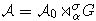be an algebraic crossed product and denote the commutant of A0 by CA(A0) = {a ∈ A | ab = ba, ∀b ∈ A0}. If the coefficient ring A0 is commutative, then I ∩ CA(A0) ≠ {0} for every non-zero two-sided ideal I in the crossed product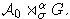As an immediate corollary to this theorem we get that, if A0 is assumed to be maximal commutative in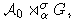then IA0 ≠ {0} for every non-zero two-sided ideal I in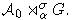Hence, in a general crossed product where the coefficient ring A0 is commutative, S1 always implies S2. As we will se in Section 2, S2 does not always imply S1.

For the convenience of the reader we shall now recall the definition and the basic properties of algebraic crossed products. For more details see e.g . Throughout this article all rings are assumed to be associative rings. Given a unital ring R we let U(R) denote the group of multiplication invertible elements of R.

Definition 1.1. A G-crossed system is a quadruple {A0, G, σ, α}, consisting of a unital ring A0, a group G (with unit element e), a map σ : G → Aut(A0) and a σ-cocycle map α : G×G → U(A0) such that for any x, y, z ∈ G and a ∈ A0 the following conditions hold:

(i) σx(σy(a)) = α(x, y) σxy(a) α(x, y)<sup>−1</sup>

(ii) α(x, y) α(xy, z) = σx(α(y, z)) α(x, yz)

(iii) α(x, e) = α(e, x) = 1A0

Let {us}S∈G be a copy (as a set) of G. Given a G-crossed system {A0, G, σ, α}, we denote by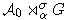G the free left A0-module having {us}S∈G as its basis and we define a multiplication on this set by

(a1 ux)(a2 uy) = a1 σx(a2) α(x, y) uxy

for all a1, a2A0 and x, y ∈ G and extend it bilinearly to all of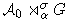. Each element of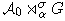G may be expressed as a formal sum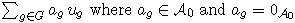for all but a finite number of g ∈ G. Explicitly, the addition and multiplication of two arbitrary elements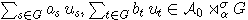is given by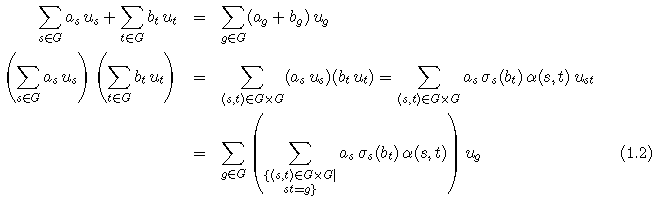Proposition 1.1 (). Let {A0, G, σ, α} be a G-crossed system. Then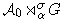is an associative unital ring (with the multiplication defined in (1.1)).

Definition 1.2. The ring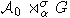is called the crossed product of the G-crossed system {A0, G, σ, α}.

The coefficient ring A0 is naturally embedded as a subring into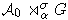via the canonical isomorphism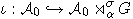defined by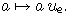Instead of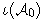we will simply write A0.

Remark 1.1. If k is a field and A is a k-algebra, then so is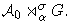Depending on the nature of the maps σ and α we will give different names to the crossed product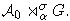If the map α is trivial, i.e α(x, y) = 1A0 for every (x, y) ∈ G × G, then we shall write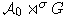and refer to it as a skew group ring. If, on the other hand, σ is trivial, i.e. σg = idA0 for every g ∈ G, then we shall write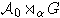and refer to it as a twisted group ring. A crossed product where both of the maps σ and α are trivial is written as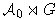and is simply refered to as a group ring.

#### Group rings, skew group rings and twisted group rings

If one wants to talk about maximal commutativity of A0, it does not really make sense unless we assume that A0 is commutative itself, so from now on we will only consider crossed products where A0 is commutative. Note that if the group G is trivial, i.e. G = {e}, then S1 and S2 are always true. In the further discussion we will therefore assume that G ≠ {e}. We will continue the further investigation by breaking it down into special cases of crossed products.

Example 2.1 (group rings). Let A0 be a unital ring and G any (non-trivial) group and denote the group ring by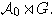Note that this corresponds to the crossed product with trivial σ and α maps. We may define the so called augmentation map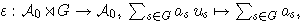and it is straightforward to check that it is in fact a ring morphism. The kernel of this map, ker(ε) is a two-sided ideal in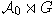and it is not hard to see that ker(ε) ∩A0 = {0}. This gives us an example of a non-zero two-sided ideal which has zero intersection with the coefficient ring A0, i.e. S2 is false. However, for each s ∈ G, us commutes with every element in A0 and hence S1 is never true for a group ring (when G ≠ {e}). In other words, in a group ring the two statements S1 and S2 are always equivalent.

For skew group rings we have the following theorems.

Theorem 2.1 (). If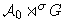is a skew group ring where the coefficient ring A0 is an integral domain and the group G is abelian, then the two assertions S1 and S2 are equivalent.

Theorem 2.2 (). Ifis a skew group ring where the coefficient ring A0 is commutative and G is a torsion-free abelian group, then the two assertions S1 and S2 are equivalent.

Remark 2.1. Note that in the previous theorems, the action σ can be trivial, but in that case the situation is already described by Example 2.1.

Example 2.2 (the algebra associated to a dynamical system). In [5,6,7] the authors studies crossed product algebras associated to dynamical systems. Suppose that we are given a nonempty set X and a bijection σ : X → X. Then (X, σ) is a discrete dynamical system where the action of n ∈ Z on x ∈ X is given by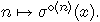By CX we denote the algebra of functions X → C under the usual pointwise operations of addition and multiplication. If we are given a subalgebra A µ CX such that it is invariant under σ and σ−1, i.e. such that if h ∈ A then h ± σ ∈ A and h ± σ−1 ∈ A, then σ induces an automorphism ˜σ : A → A defined by ˜σ(f) = f ± σ by which Z acts on A via iterations. We may now define the skew group algebra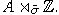In the current situation the coefficient algebra A is commutative and the group (Z, +) is clearly torsion-free and abelian, hence Theorem 2.2 is applicable. We may conclude that Theorem 2.2 is a generalization of certain parts of Corollary 4.5 in  and Theorem 4.5, Theorem 4.6, Corollary 4.7, Theorem 6.2 in .

In a twisted group ring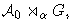just like for group rings mentioned above, the action σ is trivial and hence for each s ∈ G the element us commutes with every element in A0. In other words, A0 is never maximal commutative in a twisted group ring (when G ≠ {e}).

Example 2.3 (the field of complex numbers). Let A0 = R, G = (Z2, +) and define the cocycle α : Z2 × Z2 → R ∩ {0} by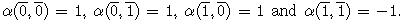It is easy to see that R oα Z2 »= C. Clearly this twisted group ring is a field and hence simple. Therefore, C is the only non-zero ideal and clearly C ∩ R ≠ {0}. However, as has already been mentioned, the coefficient ring R is not maximal commutative in C. Example 2.3 shows that in a twisted group ring, S1 may be false even though S2 is true.

#### General crossed products

A crossed product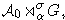where neither of the maps σ and α are trivial and hence not treated in the previous section, will be refered to as a general crossed product. For this type of crossed products we are not able to say as much as we would want to.

Theorem 3.1. If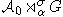is a crossed product where A0 is an integral domain, G is an abelian torsion-free group and α is such that α(s, t) = 1A0 whenever σs = idA0 or σt = idA0 , then the two assertions S1 and S2 are equivalent.

Proof. It is clear from Theorem 1.1 that S1 =) S2. Suppose that A0 is not maximal commutative. Since A0 is an integral domain, this means that there exists some s ∈ G ∩ {e} such that σs = idA0 . For arbitrary g, h ∈ G we may use condition (ii) in Definition 1.1 and the assumptions we made on α to arrive at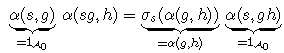and since G is abelian we get α(gs, h) = α(sg, h) = α(g, h). Let I be the two-sided ideal generated by 1A0 +us, which is an element that commutes with all of A0. The ideal I is obviously non-zero and furthermore, it is spanned by elements of the form ag ug (1A0 +us) ah uh where g, h ∈ G and ag, ah ∈ A0. We may now rewrite this expression.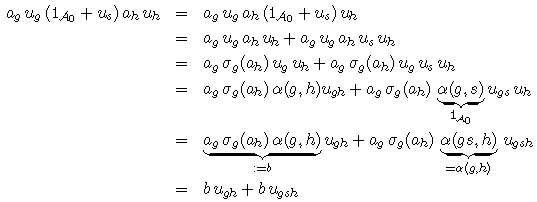Since G is abelian, it is clear that any element of I may be written in the form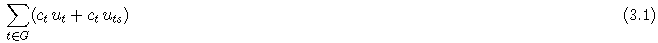for some ct ∈ A0, where t only runs over a finite subset of G. By assumtion s ≠ e and hence t ≠ ts for every t ∈ G. In particular this means that every contribution from ce to the e-graded part of the element in (3.1) comes with an equal contribution to the s-graded part. Similarly cs:s contribution to the s-graded part equals its contribution to the cs2-graded part. Furthermore, G is assumed to be torsion-free, i.e. sn ≠ e for every n ∈ Z∩ {0}, and hence the element in (3.1) can never be a non-zero element of degree e, which means I ∩ A0 = {0}. By contra positivity we conclude that S2S1 and this finishes the proof.

Remark 3.1. Note that, a twisted group ring can never fit into the conditions of Theorem 3.1, because if σ is trivial, then the conditions force α to be trivial as well.

Finite groups are clearly not torsion-free, but Example 3.1 gives an example of a situation where S1 and S2 are in fact equivalent for a general crossed product graded by a finite group. This raises the question whether or not Theorem 3.1 can be generalized to general crossed products graded by more general groups.

Example 3.1 (central simple algebras). Let A be a finite-dimensional central simple algebra over a field F. By Wedderburn’s theorem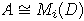where D is a division algebra over F and i is some integer. If K is a maximal separable subfield of D then [K : F] = n where [D : F] = n2. We shall assume that K is normal over F and that [A : F] = [K : F]2 (see  for motivation). Let Gal(K/F) be the Galois group of K over F. For k ∈ K and σs ∈ Gal(K/F) we shall write σs(k) for the image of k under σs. By the Noether-Skolem theorem there is an invertible element us ∈ A such that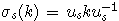for every k ∈ K. One can show that the us’s are linearly independent over K. However, the linear span over K of the us’s has dimension n2 over F, hence must be all of A. In short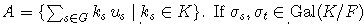and k ∈ K, then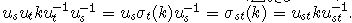This says that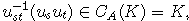in other words usut = f(s, t)ust where f(s, t) ≠ 0 is in K. Since A is an associative algebra one may verify that f : Gal(K/F) × Gal(K/F) → K ∩ {0} is in fact a cocycle. By Theorem 4.4.1 in , if K is a normal extension of F with Galois group Gal(K/F) and f is a cocycle (factor set), then the crossed product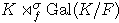is a central simple algebra over F and hence in this situation both S1 and S2 are in fact true.

#### Acknowledgement

We are grateful to Freddy Van Oystaeyen for useful discussions on the topic of this article. This work was supported by the Swedish Foundation of International Cooperation in Research and Higher Education (STINT), the Crafoord Foundation, The Royal Physiographic Society in Lund, The Swedish Royal Academy of Sciences and ”LieGrits”, a Marie Curie Research Training Network funded by the European Community as project MRTN-CT 2003-505078.

#### References

Select your language of interest to view the total content in your interested language

### Article Usage

• Total views: 12149
• [From(publication date):
September-2008 - May 25, 2019]
• Breakdown by view type
• HTML page views : 8361
• PDF downloads : 3788

## Post your commentCan't read the image? click here to refresh
###### Peer Reviewed Journals

Make the best use of Scientific Research and information from our 700 + peer reviewed, Open Access Journals

International Conferences 2019-20

Meet Inspiring Speakers and Experts at our 3000+ Global Annual Meetings

Top